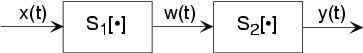# 2.5: Introduction to Systems

$$\newcommand{\vecs}{\overset { \rightharpoonup} {\mathbf{#1}} }$$ $$\newcommand{\vecd}{\overset{-\!-\!\rightharpoonup}{\vphantom{a}\smash {#1}}}$$$$\newcommand{\id}{\mathrm{id}}$$ $$\newcommand{\Span}{\mathrm{span}}$$ $$\newcommand{\kernel}{\mathrm{null}\,}$$ $$\newcommand{\range}{\mathrm{range}\,}$$ $$\newcommand{\RealPart}{\mathrm{Re}}$$ $$\newcommand{\ImaginaryPart}{\mathrm{Im}}$$ $$\newcommand{\Argument}{\mathrm{Arg}}$$ $$\newcommand{\norm}{\| #1 \|}$$ $$\newcommand{\inner}{\langle #1, #2 \rangle}$$ $$\newcommand{\Span}{\mathrm{span}}$$ $$\newcommand{\id}{\mathrm{id}}$$ $$\newcommand{\Span}{\mathrm{span}}$$ $$\newcommand{\kernel}{\mathrm{null}\,}$$ $$\newcommand{\range}{\mathrm{range}\,}$$ $$\newcommand{\RealPart}{\mathrm{Re}}$$ $$\newcommand{\ImaginaryPart}{\mathrm{Im}}$$ $$\newcommand{\Argument}{\mathrm{Arg}}$$ $$\newcommand{\norm}{\| #1 \|}$$ $$\newcommand{\inner}{\langle #1, #2 \rangle}$$ $$\newcommand{\Span}{\mathrm{span}}$$$$\newcommand{\AA}{\unicode[.8,0]{x212B}}$$

##### Learning Objectives
• Introduction to the concept of a system, which is a mechanism for manipulating signals.
• Feedback concepts and superpositions are also briefly mentioned.

Signals are manipulated by systems. Mathematically, we represent what a system does by the notation

$y(t) = S\left ( x(t) \right ) \nonumber$

with x representing the input signal and y the output signal.Figure 2.5.1 The system depicted has input x(t) and output y(t). Mathematically, systems operate on function(s) to produce other function(s). In many ways, systems are like functions, rules that yield a value for the dependent variable (our output signal) for each value of its independent variable (its input signal). The notation y(t)=S(x(t)) corresponds to this block diagram. We term S(•) the input-output relation for the system.

This notation mimics the mathematical symbology of a function: A system's input is analogous to an independent variable and its output the dependent variable. For the mathematically inclined, a system is a functional: a function of a function (signals are functions).

Simple systems can be connected together--one system's output becomes another's input--to accomplish some overall design. Interconnection topologies can be quite complicated, but usually consist of weaves of three basic interconnection forms.Figure 2.5.2 The most rudimentary ways of interconnecting systems are shown in the figures in this section. This is the cascade configuration.

The simplest form is when one system's output is connected only to another's input. Mathematically,

$w(t) = S_{1}\left ( x(t) \right )\\ y(t) = S_{2}\left ( x(t) \right ) \nonumber$

with the information contained in x(t) processed by the first, then the second system. In some cases, the ordering of the systems matter, in others it does not. For example, in the fundamental model of communication the ordering most certainly matters.

## Parallel Interconnection

A signal x(t)is routed to two (or more) systems, with this signal appearing as the input to all systems simultaneously and with equal strength. Block diagrams have the convention that signals going to more than one system are not split into pieces along the way. Two or more systems operate on x(t) and their outputs are added together to create the output y(t). Thus,

$y(t) = S_{1}\left ( x(t) \right )+ S_{2}\left ( x(t) \right ) \nonumber$

The information in x(t) is processed separately by both systems.

## Feedback Interconnection

The subtlest interconnection configuration has a system's output also contributing to its input. Engineers would say the output is "fed back" to the input through system 2, hence the terminology. The mathematical statement of the feedback interconnection is that the feed-forward system produces the output:

$y(t) = S_{1}\left ( e(t) \right ) \nonumber$

The input e(t) equals the input signal minus the output of some other system's output to:

$y(t):e(t) = x(t)- S_{2}\left ( y(t) \right ) \nonumber$

Feedback systems are omnipresent in control problems, with the error signal used to adjust the output to achieve some condition defined by the input (controlling) signal. For example, in a car's cruise control system, x(t) is a constant representing what speed you want, and y(t) is the car's speed as measured by a speedometer. In this application, system 2 is the identity system (output equals input).

This page titled 2.5: Introduction to Systems is shared under a CC BY 1.0 license and was authored, remixed, and/or curated by Don H. Johnson via source content that was edited to the style and standards of the LibreTexts platform; a detailed edit history is available upon request.